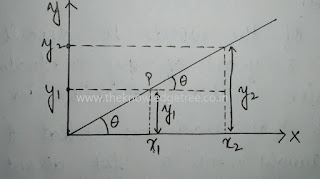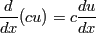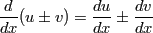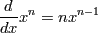## Mathematical tools everyone should know

Graph: It is the fictional representations of variation of one quantity with respect to another quantity. Shape of graph depends on the relation between two variables.
standard equation came from graph, its prove discuss later in math and link will be updated here.
y = mx  where  'm' is slope of straight  line.fig.1.
from the above fig.1.,  m = tanθ = [(y2 -y1)/(x2-x1)]
tanθ  = (Δy/Δx)

Differentiation: change in a function w.r.t. another function.

If we draw a graph for function,the derivative at a certain point corresponds to the 'slope' or 'gradient' of the function at that point as in fig.2.fig.2.

Consider a continuous curve y=f(x) and P be a point on this curve.If tangent to the curve at P makes an angle with the x-axis then at point P
dy/dx= tanθ = m
Case 1:If dy/dx
then the tangent makes an acute angle with x-axis as in fig.3.fig.3.

Case 2:If dy/dx
then the tangent makes an obtuse angle with x-axis as in fig.4.fig.4.

Case 3:If dy/dx

then the tangent is parallel to x-axis as in fig.5.fig.5.

Rules for differentiation:

1(a). Derivative of a constant function is zero.
i.e. dy/dx(k)=0
(b). Let c is a constant and f(x) is a differentiable function then
i.e.2. If two functions are given then rename

(a).(b).                                      (product rule)

(c).
provided v≠0           (quotient rule)

3. Derivative of a function of function                    (Chain rule)

If y is differentiable function of 'u and u' is differentiable function of 'x' then

Some important resultsIntegration: Anti derivative or Inverse of differentiation.
Standard integral formulae:

where c is constant.

References: NCERT books, Moderns pub.,mathisfunforum.com Internet Sources

—vvv —

#### 1 comment:

1.